# Kleene-Mostowski classification

Jump to: navigation, search

A classification of number-theoretic predicates, introduced independently by S.C. Kleene  and A. Mostowski . The class of all recursive predicates is denoted simultaneously by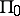and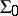. For each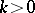the classis defined as the class of all predicates expressible in the form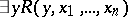, where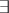is the existential quantifier andis a predicate in the class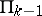, while the class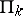is defined as the class of predicates expressible in the form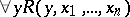, where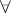is the universal quantifier and the predicate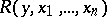belongs to the class. In this way a double sequence of classes is obtained: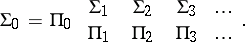If a predicate belongs toor, then it belongs to the classes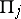and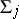for any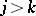, that is,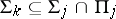and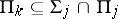for any. If, then there exist predicates innot belonging toand also predicates innot belonging to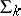, that is,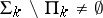and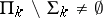. A predicate belongs to one of the classesorif and only if it is expressible in the language of formal arithmetic (cf. Arithmetic, formal). If a predicate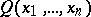belongs to(or) then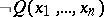, whereis the negation sign, belongs to(respectively,). A predicateis recursive (cf. Recursive predicate) if and only ifandbelong to, i.e.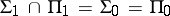. If, then.

The classification of sets defined in the language of formal arithmetic is based on the classification of predicates: A setbelongs to(or) if the predicate "x M" belongs to this class.